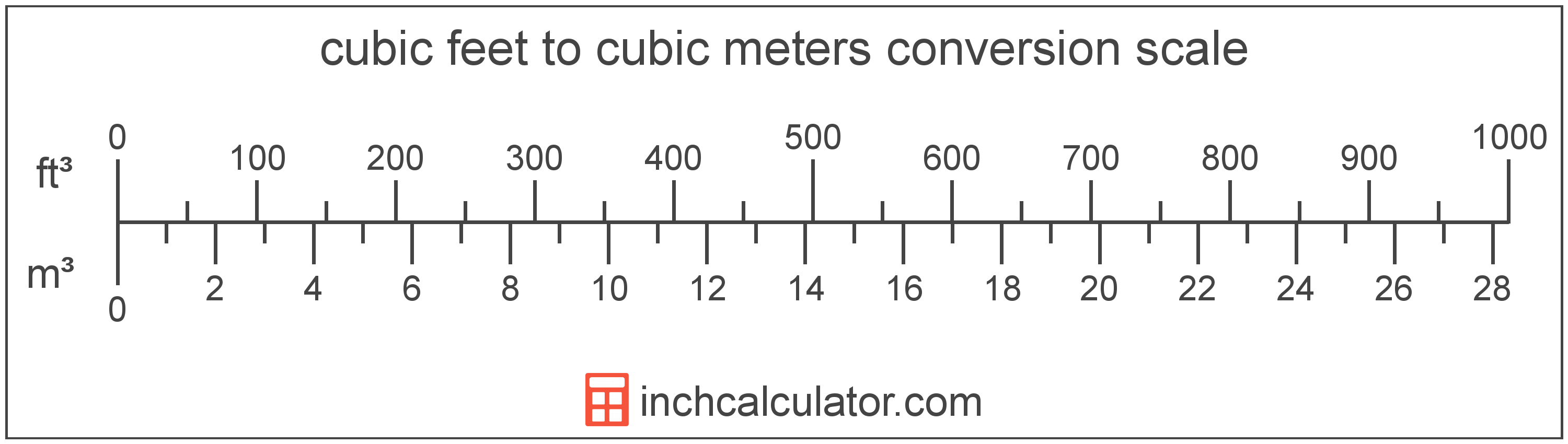# Cubic Feet to Cubic Meters Conversion

Enter the volume in cubic feet below to get the value converted to cubic meters.

(find cubic feet)
Results in Cubic Meters:1 ft³ = 0.028317 m³

## How to Convert Cubic Feet to Cubic Meters

To convert a cubic foot measurement to a cubic meter measurement, multiply the volume by the conversion ratio.

Since one cubic foot is equal to 0.028317 cubic meters, you can use this simple formula to convert:

cubic meters = cubic feet × 0.028317

The volume in cubic meters is equal to the cubic feet multiplied by 0.028317.

For example, here's how to convert 5 cubic feet to cubic meters using the formula above.
5 ft³ = (5 × 0.028317) = 0.141584 m³## Cubic Feet

The cubic foot is a unit of volume that is equal to the space consumed by a cube having sides one foot on each edge.

The cubic foot is a US customary and imperial unit of volume. A cubic foot is sometimes also referred to as a cubic ft. Cubic feet can be abbreviated as ft³, and are also sometimes abbreviated as cu foot, cu ft, or CF. For example, 1 cubic foot can be written as 1 ft³, 1 cu foot, 1 cu ft, or 1 CF.

Try our cubic feet calculator to calculate the volume of a space.

## Cubic Meters

One cubic meter is equal to the volume of a cube with each edge measuring one meter.

The cubic meter, or cubic metre, is the SI derived unit for volume in the metric system. Cubic meters can be abbreviated as , and are also sometimes abbreviated as cu m, CBM, cbm, or MTQ. For example, 1 cubic meter can be written as 1 m³, 1 cu m, 1 CBM, 1 cbm, or 1 MTQ.

Try our cubic meters calculator to calculate the volume of a space.

## Cubic Foot to Cubic Meter Conversion Table

Cubic foot measurements converted to cubic meters
Cubic Feet Cubic Meters
1 ft³ 0.028317 m³
2 ft³ 0.056634 m³
3 ft³ 0.084951 m³
4 ft³ 0.113267 m³
5 ft³ 0.141584 m³
6 ft³ 0.169901 m³
7 ft³ 0.198218 m³
8 ft³ 0.226535 m³
9 ft³ 0.254852 m³
10 ft³ 0.283168 m³
11 ft³ 0.311485 m³
12 ft³ 0.339802 m³
13 ft³ 0.368119 m³
14 ft³ 0.396436 m³
15 ft³ 0.424753 m³
16 ft³ 0.45307 m³
17 ft³ 0.481386 m³
18 ft³ 0.509703 m³
19 ft³ 0.53802 m³
20 ft³ 0.566337 m³
21 ft³ 0.594654 m³
22 ft³ 0.622971 m³
23 ft³ 0.651287 m³
24 ft³ 0.679604 m³
25 ft³ 0.707921 m³
26 ft³ 0.736238 m³
27 ft³ 0.764555 m³
28 ft³ 0.792872 m³
29 ft³ 0.821189 m³
30 ft³ 0.849505 m³
31 ft³ 0.877822 m³
32 ft³ 0.906139 m³
33 ft³ 0.934456 m³
34 ft³ 0.962773 m³
35 ft³ 0.99109 m³
36 ft³ 1.0194 m³
37 ft³ 1.0477 m³
38 ft³ 1.076 m³
39 ft³ 1.1044 m³
40 ft³ 1.1327 m³